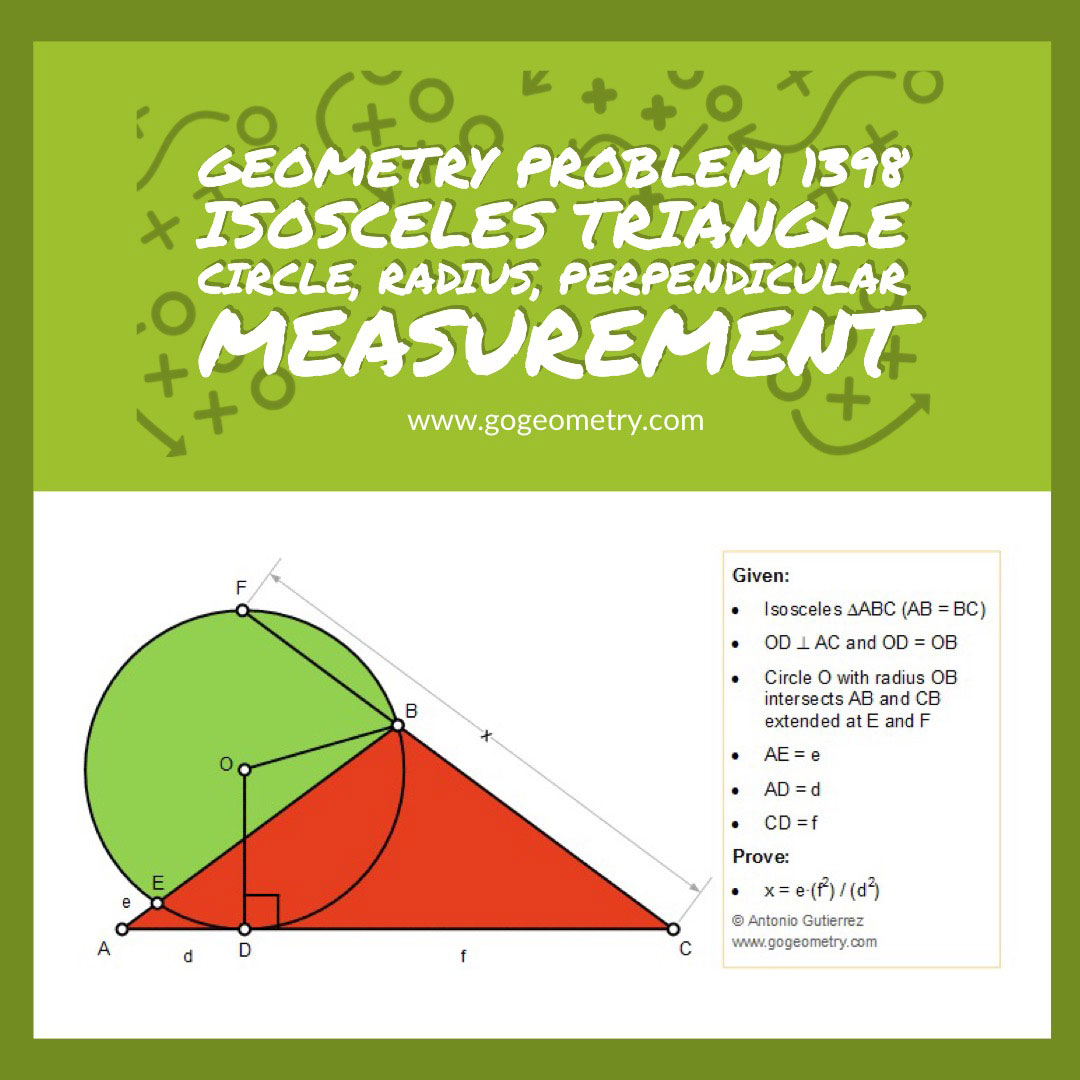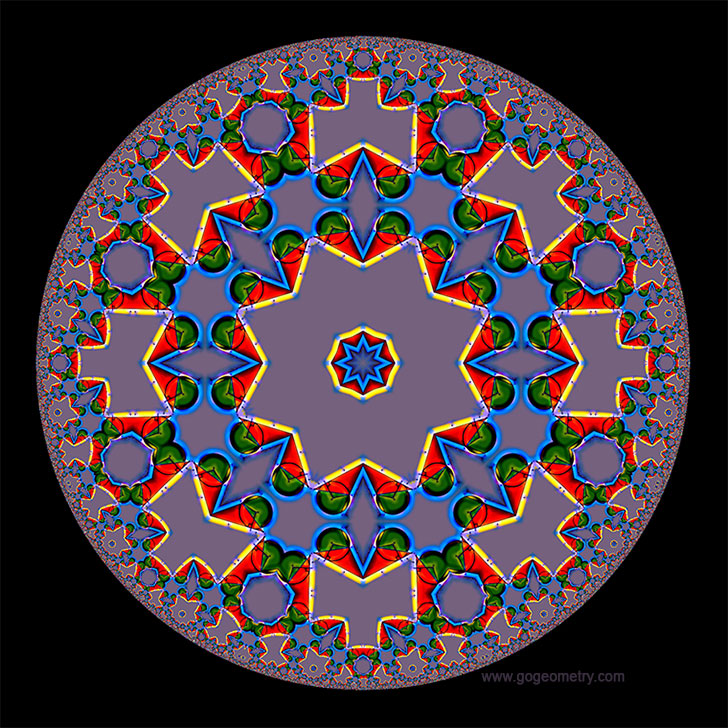Geometry Problem 1398: Isosceles Triangle, Circle, Radius, Perpendicular, Measurement

Proposition

The figure below shows an isosceles triangle ABC (AB = BC). D is a point on AC and line DO is perpendicular to AC so that OD = OB. The circle with radius OB intersects AB and CB extended at E and F, respectively. If AE = e, AD = d, and CD = f, prove that x = e.(f2)/(d2)Poster of Problem 1398 using iPad AppsGeometric Art: Hyperbolic Kaleidoscope of problem 1398 using iPad AppsGeometric Art using Mobile Apps

Geometric art is a form of art based on the use and application of geometric figures. A geometric figure is any set or combination of points, lines, surfaces and solids. A mobile app or mobile application software is a computer program designed to run on smartphones and tablet computers.

Hyperbolic Kaleidoscope, Poincare Disk Model

Poincare disk model is a model of 2-dimensional hyperbolic geometry in which the points of the geometry are inside the unit disk, and the straight lines consist of all segments of circles contained within that disk that are orthogonal to the boundary of the disk, plus all diameters of the disk. Read more.